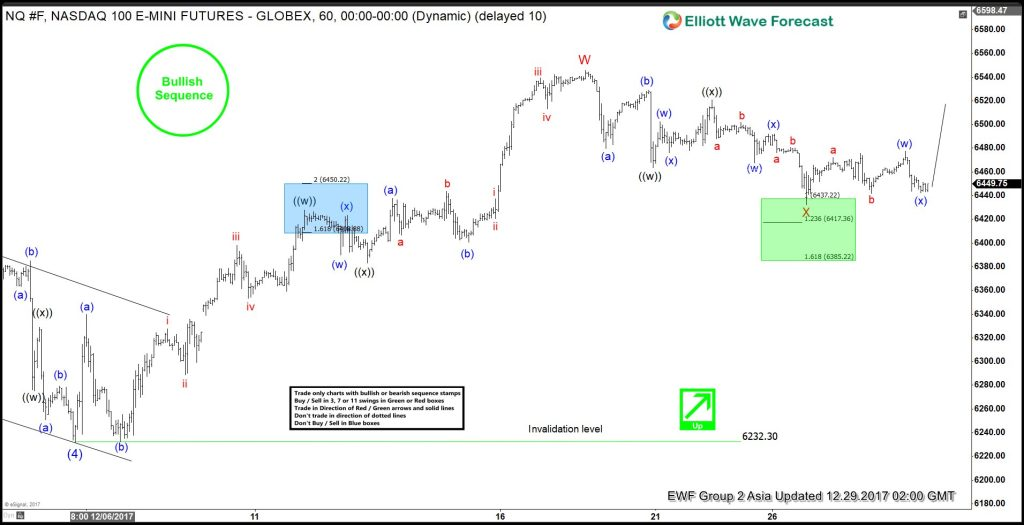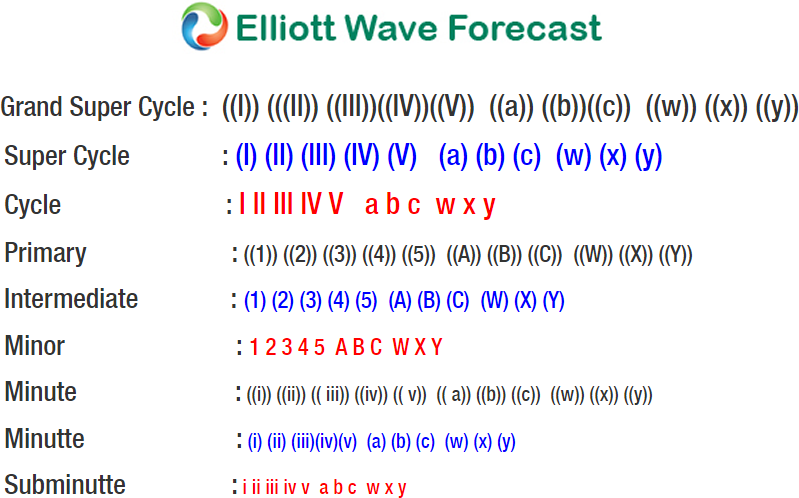# Nasdaq Elliott Wave Analysis: More Upside Favored While Above 6232.3

Nasdaq Short Term Elliott Wave view suggests that the Index remains bullish as far as pullbacks stay above Intermediate wave (4) at 6232.3. Rally from Intermediate wave (4) low unfolded as a double three Elliott Wave structure where Minor wave W ended at 6545.75 and Minor wave X is proposed complete at 6432.25 in the green box. Internal of Minor wave W unfolded as a double three Elliott Wave structure where Minute wave ((w)) ended at 6427.75, Minute wave ((x)) ended at 6383, and Minute wave ((y)) of W ended at 6545.75.

Internal of Minor wave X unfolded as a double three Elliott Wave structure where Minute wave ((w)) ended at 6463.25, Minute wave ((x)) ended at 6520.75, and Minute wave ((y)) of X ended at 6432.25. Near term, while dips stay above 6432.25, but more importantly as far as pivot at 12/5 low (6232.3) stays intact, expect Index to extend higher. Unless already long with a risk free trade from the green box area, we prefer to wait for the Index to break above Minor wave W at 6545.75 before buying the dips again. Until the Index breaks above Minor wave W at 6545.75, a double correction in Minor wave X still can’t be ruled out.

If the Index breaks below 12/26 low (6432.3) from here, then it could either form a triple three or double three correction from 12/19 peak. In the case of a triple three correction, Index should then extend lower to 6371 – 6391 area to end Minor wave X before the rally resumes. In the case of a double three correction, then Index can go to as low as 6234 – 6361 to end Minor wave X before buyers appear for at least a 3 waves bounce.

#### Nasdaq 1 Hour Elliott Wave Chart